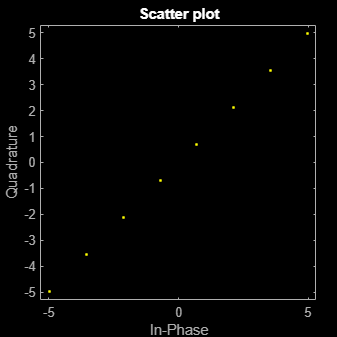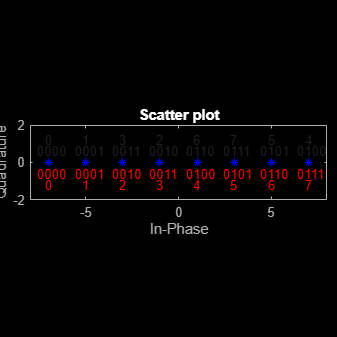# pammod

Pulse amplitude modulation (PAM)

## Syntax

``y = pammod(x,M)``
``y = pammod(x,M,initphase)``
``y = pammod(x,M,initphase,symorder)``

## Description

````y = pammod(x,M)` returns the complex envelope of the modulation of the input message signal, `x`, using PAM and the alphabet size, `M`.```

example

````y = pammod(x,M,initphase)` specifies the initial phase of the modulated signal.```

example

````y = pammod(x,M,initphase,symorder)` specifies binary-coded or Gray-coded mapping order for the modulation symbols.```

## Examples

collapse all

Generate random data symbols and apply pulse amplitude modulation.

Set the modulation order.

`M = 8;`

Generate random integers and apply PAM modulation having an initial phase of $\pi$/4.

```data = randi([0 M-1],100,1); modData = pammod(data,M,pi/4);```

Display the PAM constellation diagram.

`scatterplot(modData)`Plot PAM symbol mapping for Gray-coded and binary-coded data.

Set the modulation order, and then create a data sequence containing a complete set of constellation points.

```M = 8; data = 0:M-1;```

Modulate and demodulate Gray and natural binary encoded data.

```symgray = pammod(data,M,0,'gray'); mapgray = pamdemod(symgray,M,0,'gray'); symbin = pammod(data,M,0,'bin'); mapbin = pamdemod(symbin,M,0,'bin');```

Plot the constellation points using one of the symbol sets. For each constellation point, assign a label indicating the Gray-coded and binary-coded values for each symbol.

• For Gray-code symbol mapping, adjacent constellation points differ by a single bit and are not numerically sequential.

• For binary-coded mapping, adjacent constellation points follow the binary encoding and are sequential.

```scatterplot(symgray,1,0,'b*'); for k = 1:M text(real(symgray(k))-0.6,imag(symgray(k))+0.6,... dec2base(mapgray(k),2,4)); text(real(symgray(k))-0.2,imag(symgray(k))+1.2,... num2str(mapgray(k))); text(real(symbin(k))-0.6,imag(symbin(k))-0.6,... dec2base(mapbin(k),2,4),'Color',[1 0 0]); text(real(symbin(k))-0.2,imag(symbin(k))-1.2,... num2str(mapbin(k)),'Color',[1 0 0]); end axis([-M M -2 2])```## Input Arguments

collapse all

Input signal, specified as a vector or matrix of integers in the range of [0, `M` – 1].

Example: `randi([0 3],100,1)`

Data Types: `double`

Modulation order, specified as a power of two.

Example: `4`

Data Types: `double`

Initial phase of the modulated signal (in radians), specified as a real scalar.

Example: `pi/4`

Data Types: `double`

Mapping order for the modulation symbols, specified as `'bin'` or `'gray'`. This argument specifies how the function assigns binary vectors to corresponding integers.

• If `symorder` is `'bin'`, the function uses a binary-coded mapping order.

• If `symorder` is `'gray'`, the function uses a Gray-coded mapping order.

Data Types: `char` | `string`

## Output Arguments

collapse all

Complex baseband representation of a PAM-modulated signal, returned as vector or matrix of complex values. The modulated signal has a minimum Euclidean distance of 2. The columns of `y` represent independent channels.

Data Types: `double` | `single`
Complex Number Support: Yes

## Version History

Introduced before R2006a